### Home > INT3 > Chapter 9 > Lesson 9.2.2 > Problem9-114

9-114.
1. For the polynomial function f(x) = x3 – 5x2 + 11x – 15, which of the following expressions are possible factors? Homework Help ✎

1. (x + 1)

2. (x – 2)

3. (x + 4)

4. (x – 3)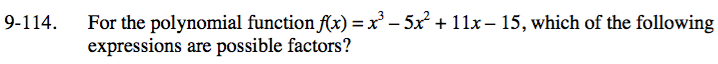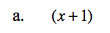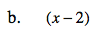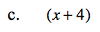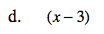The factored form of f(x) will be (x ± a)(x ± b)(x ± c).
What must be true about the product of a, b, and c?